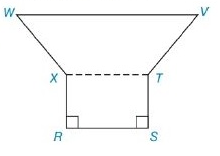Chapter 8.2, Problem 28EElementary Geometry For College St...

7th Edition
Alexander + 2 others
ISBN: 9781337614085

Solutions

Chapter
SectionElementary Geometry For College St...

7th Edition
Alexander + 2 others
ISBN: 9781337614085
Textbook Problem

In Exercises 27 to 30, find the area of the figure shown. Given: Hexagon RSTVWXwith W V - ∥ X T - ∥ R S - R S = 10 S T = 8 T V = 5 W V = 16 W X - ≅ V T - Find: A R S T V W XTo determine

To Find:

Area of the hexagon provided.

Explanation

Formula Used:

Split the hexagon into rectangle and trapezium and find the corresponding areas and sum it up.

Pythagorean theorem for the right angle triangle ABC for the hypotenuse AC,

AC2=AB2+BC2.

Area of rectangle =l×b

Area of trapezium =12h(b1+b2)

Calculation:

Let the vertices of the provided hexagon be R, S,T, V, W and X.

Let’s divide the quadrilateral into a rectangle and a trapezium. Draw a line from the point X to T.

Here RSTX forms a rectangle, the remaining XTVW forms the trapezium.

It is given that RS=10, ST=8, TV=5, WV=16 and WX-VT-.

Consider the rectangle TX,

Area of the rectangle RSTX is l×b=RS×ST=10×8=80 sq. units.

Consider the trapezium XTVW,

It is given that WX-VT-. The given trapezium is an isosceles trapezium.

Since RSTX is a rectangle, RS=TX=10

Still sussing out bartleby?

Check out a sample textbook solution.

See a sample solution

The Solution to Your Study Problems

Bartleby provides explanations to thousands of textbook problems written by our experts, many with advanced degrees!

Get Started

Solve the equations in Exercises 126. x+4x+1+x+43x=0

Finite Mathematics and Applied Calculus (MindTap Course List)

For the following scores, find the value of each expression: X 3 2 4 2 a. X b. (X)2 c. X 2 d. (X 2)

Essentials of Statistics for The Behavioral Sciences (MindTap Course List)

Construct a drawing of the fourth hexagonal number.

Mathematical Excursions (MindTap Course List)

True or False: The graph at the right is the graph of a function.

Study Guide for Stewart's Single Variable Calculus: Early Transcendentals, 8th